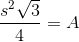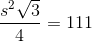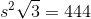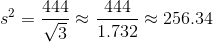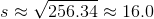# PSAT Math : How to find the length of an edge of a tetrahedron

## Example Questions

### Example Question #1 : Tetrahedrons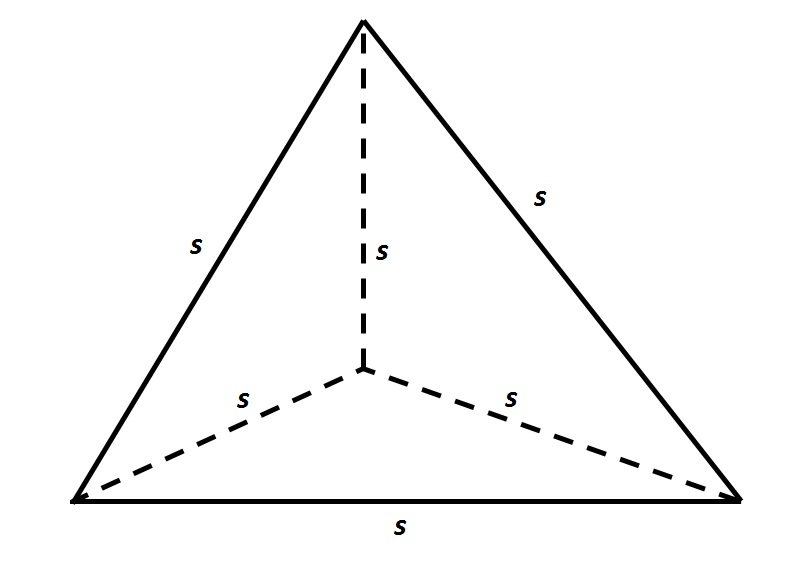Refer to the above tetrahedron, or four-faced solid. The surface area of the tetrahedron is 444. Evaluateto the nearest tenth.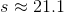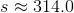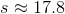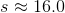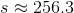Explanation:

The tetrahedron has four faces, each of which is an equilateral triangle with sidelength. Since the total surface area is 444, each triangle has area one fourth of this, or 111. To find, set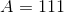in the formula for the area of an equilateral triangle: